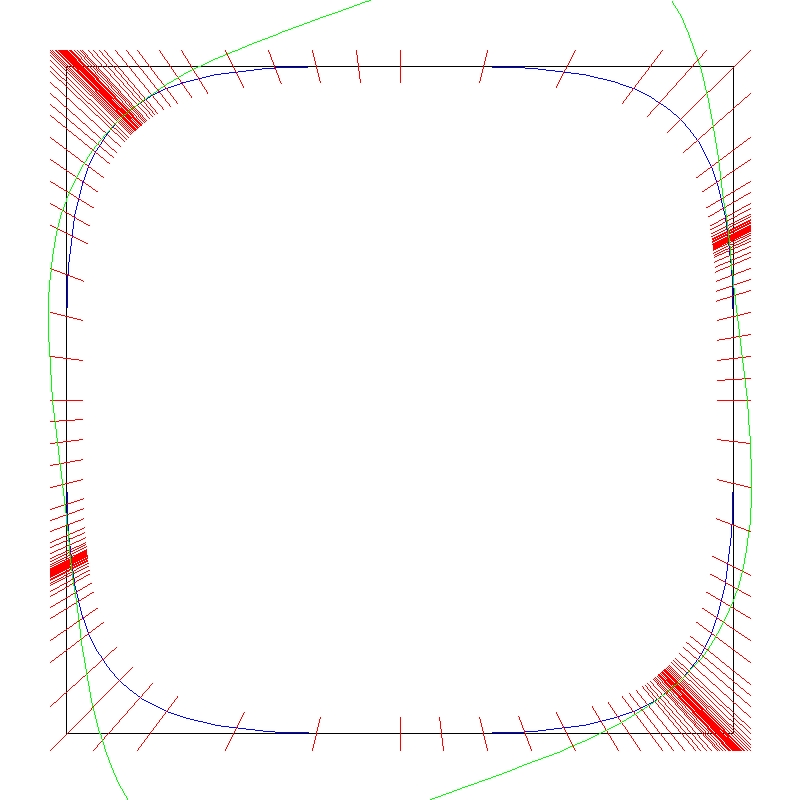# A Counterexample to a Conjecture of Matsaev

This page is written in support of an article entitled "A Counterexample to a Conjecture of Matsaev" published in Linear Algebra and its Applications 435 (2011), pp. 323-329. The question that the article addresses is the following one:

If T is a linear contraction on an L^p space (for 1 \leq p \leq \infty) and P is a polynomial in one variable, then \|P(T)\| \leq \|P(S)\| where S is the unilateral shift on \ell^p.

We show that this question has a negative answer for p=4 and, in fact, the situation is as bad as it can possibly be. The question has an affirmative answer if T is a scalar or if P is a degree one polynomial. In the paper we show that it fails in general if p=4, T is a 2 by 2 matrix (i.e. an operator on a 2-dimensional L^4 space) and P is a quadratic polynomial.

We assume below that the reader is familiar with the LAA article. It is not our objective to reproduce the article here. Our objectives are:

• To provide a Maple computer program which will verify claims made in the article so that a reader may verify the result for himself/herself.
• To provide some computational tools for evaluating the norms of operators from low-dimensional \ell^p spaces to general Banach spaces with easily computable norms.

### The Maple Verification Program.

To verify the results of the article completely, it is necessary to show that two matrices with rational entries have \ell^4 operator norm bounded by unity. One of these matrices is 2 by 2 and the other is 7 by 5. According to the depth first variant of the algorithm given in the article, this is achieved in the Maple worksheet below. The code has been written in such a way as to avoid the use of floating point numbers. All the calculations are done with rational numbers which Maple handles with arbitrarily high precision. Therefore, there is no roundoff error whatsoever in this program. Using Maple and writing the code in this tortured way naturally leads to long running times. However, the code completes on a modern computer in under 24 hours.

There is no documentation in the program itself. The documentation, such as it is, is to be found in the accompanying PDF file.The diagram above shows the surface subcubes and the patches that figure in the computation for the 2 by 2 matrix. The black square is the unit sphere of \ell^\infty, the blue curve is the unit sphere of \ell^4, the red lines mark the ends of the surface subcubes and patches that are successfully eliminated. Finally, the green curve represents the set of vectors v such that \|Tv\|_4 = 1 where T denotes the matrix under consideration. We perceive that where the blue and green curves are close, the patches have to be much smaller to achieve elimination. It's not obvious at this degree of magnification, but in fact, the green curve lies entirely outside the blue one.

### Computational Tools

We provide C++ code to evaluate the norm of an operator from a low-dimensional \ell^p space with p \geq 2 to a general Banach spaces with easily computable norm. The code is presented as a Microsoft Visual C++ 2008 Express Edition project. This IDE is available for free download from the Microsoft Corporation. The project is set up to compute an upper bound for the \ell^4 operator norm of the 7 by 5 matrix referred to above. The arithmetic is double precision floating point and roundoff errors are ignored, but unlikely to be significant. The program runs on a modern PC in about 5 seconds.